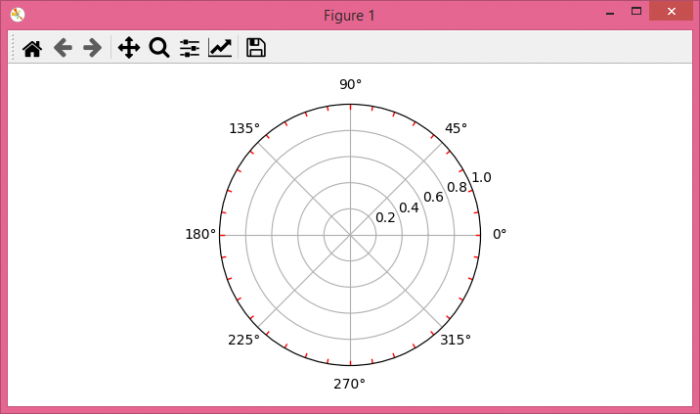# How to create minor ticks for a polar plot in matplotlib?

To create minor ticks for a polar plot in matplotlib, we can take the following steps

## Steps

• Set the figure size and adjust the padding between and around the subplots.

• Create r (radius) and theta data points using numpy.

• Add a subplot to the current figure.

• Iterate the points between 0 to 360 with step=10 and plot them to get the ticks.

• To display the figure, use Show() method.

## Example

import numpy as np
import matplotlib.pyplot as plt

# Set the figure size
plt.rcParams["figure.figsize"] = [7.00, 3.50]
plt.rcParams["figure.autolayout"] = True

# radius and theta for the polar plot
r = np.arange(0, 5, 0.1)
theta = 2 * np.pi * r

ax = plt.subplot(111, projection='polar')

tick = [ax.get_rmax(), ax.get_rmax() * 0.97]

# Iterate the points between 0 to 360 with step=10
for t in np.deg2rad(np.arange(0, 360, 10)):
ax.plot([t, t], tick, lw=1, color="red")

# Display the plot
plt.show()


## Output

It will produce the following output −